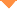首页 > 论文 > 红外与激光工程 > 48卷 > 5期(pp：517001--1)

Precise capacitance measurement by laser locking Fabry-Perot interferometer

• 摘要• 论文信息• 参考文献• 被引情况• PDF全文Abstract

In order to realize precise measurement for pF level capacitor, a capacitance measurement system based on laser locking Fabry-Perot interferometer was established and its basic principle, method of interferometer locking and determination of order numbers variation were studied. Firstly, according to the Lampard and Thompson electrical theorem, configurations and operation modes of Fabry-Perot interferometer were presented, and a displacement measurement by locking interferometer was demonstrated.Then the optical layout for Fabry-Perot interferometer was presented, and the operation status of the interferometer locking servo system and the fluctuation of cavity length in close loop were carefully analyzed. After the feasibility of determining the order numbers through the prior value of 1 pF standard capacitor was evaluated, the experiment process for confirming the variation of order numbers was presented. Finally, the character of step moving displacement and measurement repeatability of 1 pF standard capacitor were discussed. Experimental results indicate that the peak to peak fluctuation of the interferometer is 0.4 nm after its locking, and the repeatability of 1 pF standard capacitor measurement is 5.0×10-9. It can satisfy the requirements of precisely measuring the pF level capacitor. However, further study of the displacement measurement will focus on the uncertainty evaluation and optimization for the displacement measurement system.【1】Thompson A M, Lampard D G. A new theorem in electrostatics and its application to calculable standards of capacitance [J]. Nature, 1956, 177(455): 888.

【2】Thompson A M. The precise measurement of small capacitances [J]. IRE Trans Instrum, 1958, 7(3): 245-253.

【3】Lu Z L, Huang L, Yang Y, et al. Research for reproducing SI unit of capacitance by the calculable capacitor with a movable guard rod [J]. Acta Metrologica Sinica, 2014, 35(6): 521-527.

【4】Thompson A M. The cylindrical cross-capacitor as a calculable standard [C]//IEEE Transactions on Instrumentation and Meansurement, 1959, 106(27): 307-310.

【5】Lampard D G, Cutkosky R D. Some results on the cross-capacitances per unit length of cylindrical three-terminal capacitors with thin dielectric films on their electrodes [C]// Proc IEE - Part C: Monographs, 1960, 107(11): 112-119.

【6】Makow D, Campbell J B. Circular four electrode capacitors for capacitance standards [J]. Metrologia, 1972, 8(4): 148-155.

【7】Rayner G H. NPL calculable capacitor[J]. IEEE Transactions on Instrumentation and Meansurement, 1972, 21(4): 361-365.

【8】Cutkosky R D. New NBS measurements of the absolute farad and ohm [C]//IEEE Transactions on Instrumentation and Meansurement, 1974, 23(4): 305-309.

【9】Cross Capacitor Group of National Institute of Metrology. A calculable cross capacitor and absolute measurement of electrical impedance [J]. Acta Metrologica Sinica, 1980(1): 16-26.

【10】Jones K, Corney A C. The PEL calculable capacitor[J]. Metrologia, 1987, 24(1): 1-11.

【11】Small G W. Refinements to the use of the NML calculable capacitor in relation to SI ohm determinations [C]//Conf Precis Electromagn Meas (CPEM), 1996: 8-9.

【12】Trapon G, Thevenot O, Lacueille J C, et al. Realization of the farad at BNM-LCIE [C]//Conf Precis Electromagn Meas(CPEM), 1998: 448-449.

【13】Small G W, Fiander J R. Design of a calculable cross-capacitor [C]//Conf Precis Electromagn Meas (CPEM), 2004: 485-486.

【14】Thevenot O, Lahousse L, Consejo C, et al. Towards a determination of RK in terms of the new LNE calculable cross capacitor [C]//Proc Simposio de Metrologia, Santiago de Querétaro, 2008, 1142: 1-7.

【15】Small G W, Fiander J R. Fabrication and measurement of the main electrodes of the NMIA-BIPM calculable cross capacitors [J]. IEEE Transactions on Instrumentation and Meansurement, 2011, 60(7): 2489-2494.

【16】Yicheng W, Pratt J, Rae Duk L, et al. Main electrodes alignment for NIST calculable capacitor [C]//Conf Precis Electromagn Meas(CPEM), 2012: 392-393.

【17】Lu Z, Huang L, Yang Y, et al. An initial reproduction of SI capacitance unit from a new calculable capacitor at NIM[J].IEEE Transactions on Instrumentation and Meansurement, 2015, 64(6): 1496-1502.

【18】Wang J, Qian J, Liu Z, et al. Displacement determination of the guard electrode for the new calculable capacitor at NIM [C]//IEEE Transactions on Instrumentation and Measurement, 2016, 65(11): 2569-2577.

【19】Yicheng W, Rae Duk L, Liang L, et al. Next-generation calculable capacitor using a tunable-laser interferometer [C]// Conf Precis Electromagn Meas (CPEM), 2008: 686-687.

【20】Lawall J R. Fabry-Perot metrology for displacements up to 50 mm [J]. J Opt Soc Am A, 2005, 22(12): 2786-2798.

【21】Durand M, Lawall J, Wang Y. Fabry-Perot displacement interferometry for next-generation calculable capacitor[J].IEEE Transactions on Instrumentation and Meansurement, 2011, 60(7): 2673-2677.

【22】Wang Y, Yu Y, Pratt J R. Evaluation of a continuously variable calculable capacitor[J]. IEEE Transactions on Instrumentation and Meansurement, 2017, 66(6): 1503-1510.

【23】Fletcher N, Goebel R, Robertsson L, et al. Progress towards a determination of RK at the BIPM [C]//Conf Precis Electromagn Meas(CPEM), 2012: 252-253.

【24】Lampard D G. A new theorem in electrostatics with applications to calculable standards of capacitance [J]. IEE -Part C: Monographs, 1957, 104(6): 271-280.

【25】Wang J B, Qian J, Liu Z Y, et al. Methode of phase correction of displacement measurement using Fabry-Perot interferometer in calculable capacitor [J]. Acta Physica Sinica, 2016, 65(11): 110601.

Wang Jianbo,Qian Jin,Yin Cong,Lu Zuliang,Huang Lu,Yang Yan. Precise capacitance measurement by laser locking Fabry-Perot interferometer[J]. Infrared and Laser Engineering, 2019, 48(5): 0517001